# Factorising examples

Example 1

Factor the number 12

Write down the number and put two ladders up against it.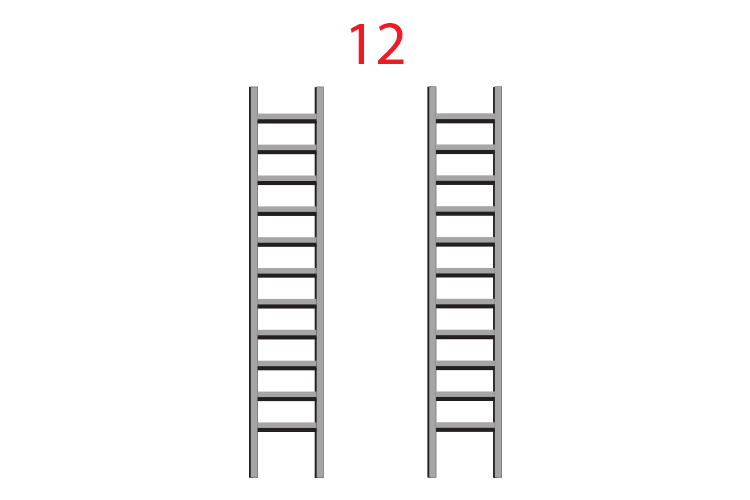Then write the numbers from 1 down the left ladder.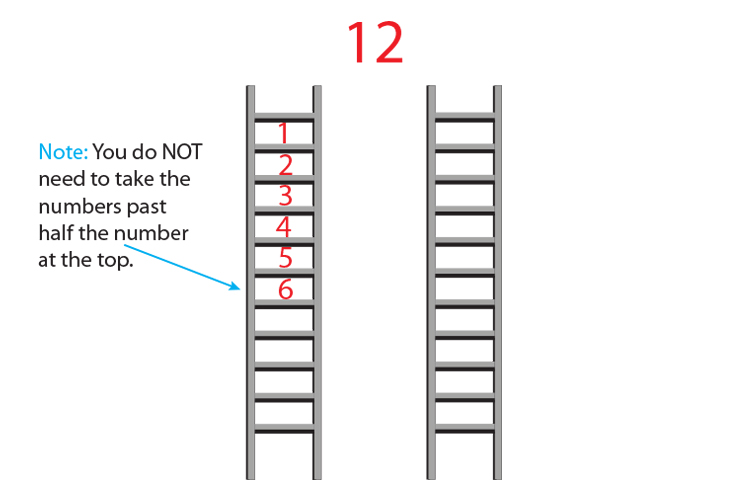Now fill the right ladder with the numbers that multiply to give 12.Therefore the factors of 12 are:

1 and 12

2 and 6

3 and 4

Alternatively the factors of 12 are 1, 2, 3, 4, 6 and 12

Example 2

Factor the number 15

Therefore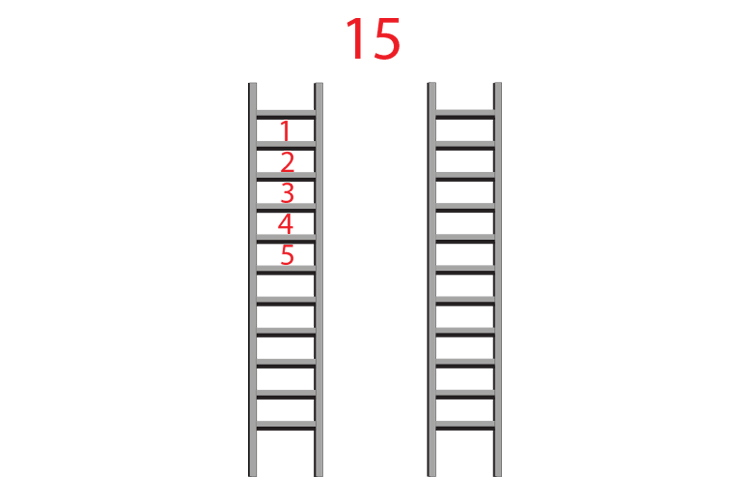And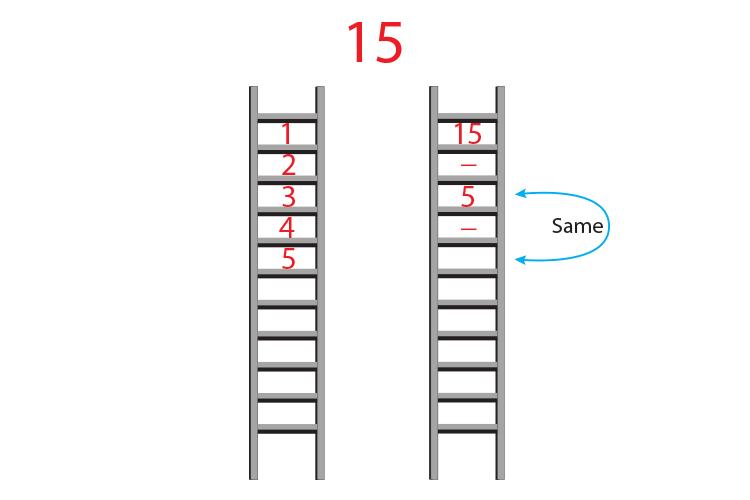Therefore the factors of 15 are:

1 and 15

3 and 5

Alternatively the factors of 15 are 1, 3, 5 and 15.

Example 3

Factor the number 6

Therefore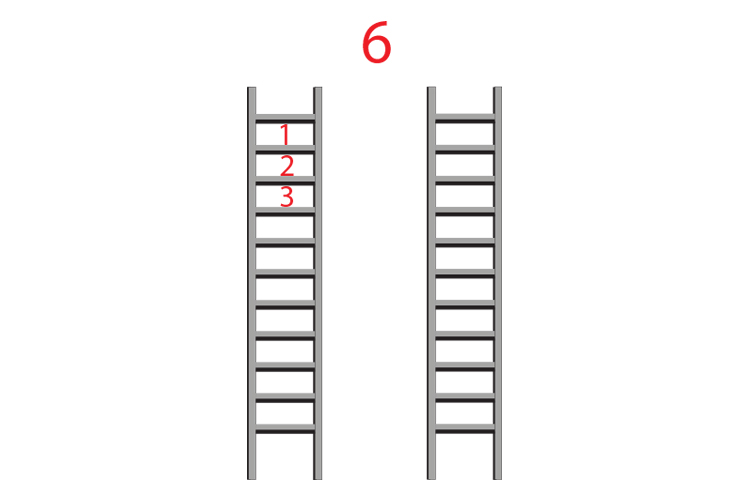And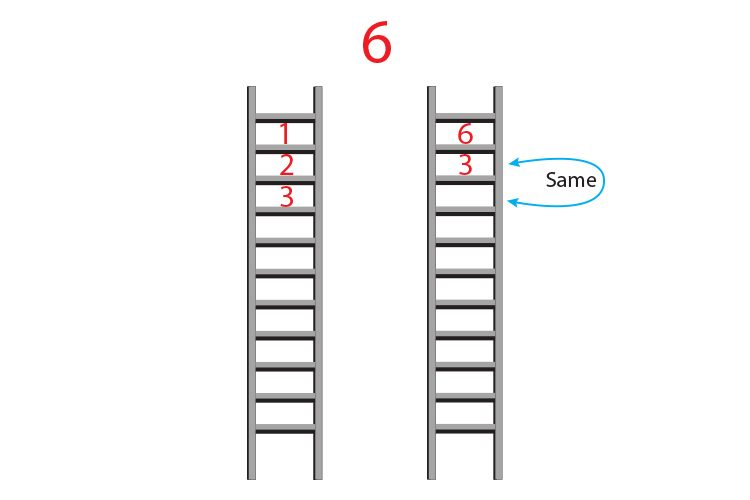Therefore the factors of 6 are:

1 and 6

2 and 3

Alternatively the factors of 6 are: 1, 2, 3 and 6.